## bi_baby_halleyBirthday: 08/17

## Contact

• Add to Friends
• Send Message

## Interest Tags

### Hobbies/Interests

hey sexies wink
i was love_em_pussys but my account was banned for being too naughty but what can i say? i just cant help my self smile
so anyways ... i love to cyber
i love to rp ! my favorites are student-teacher, nurse-patient smile
i loved to be raped <3 all situations smile
im bi but i prefer the ladies but i make exceptions smile

im always horny and im really fun smile just ask around wink
so drop me a pm so we can "talk" smile

## Media

Unable to identify Vimeo video URL.

hey girls and guys ive been getting a ton of pms and comments lately and sometimes i forget one of yours or get confused, so if i dont respond in a while, just send it again and ill be sure to continue anything thats going on wink

## love me

<3<3<3<3<3<3<3<3<3<3<3<3<3<3<3<3<3<3<3<3<3<3
<3<3<3<3<3<3<3<3<3<3<3<3<3<3<3<3<3<3<3<3<3<3
<3<3<3<3<3<3<3<3<3<3<3<3<3<3<3<3<3<3<3<3<3<3
<3<3<3<3<3<3<3<3<3<3<3<3<3<3<3<3<3<3<3<3<3<3
<3<3<3<3<3<3<3<3<3<3<3<3<3<3<3<3<3<3<3<3<3<3
<3<3<3<3<3<3<3<3<3<3<3<3<3<3<3<3<3<3<3<3<3<3
<3<3<3<3<3<3<3<3<3<3<3<3<3<3<3<3<3<3<3<3<3<3
<3<3<3<3<3<3<3<3<3<3<3<3<3<3<3<3<3<3<3<3<3<3
<3<3<3<3<3<3<3<3<3<3<3<3<3<3<3<3<3<3<3<3<3<3
<3<3<3<3<3<3<3<3<3<3<3<3<3<3<3<3<3<3<3<3<3<3
<3<3<3<3<3<3<3<3<3<3<3<3<3<3<3<3<3<3<3<3<3<3
<3<3<3<3<3<3<3<3<3<3<3<3<3<3<3<3<3<3<3<3<3<3
<3<3<3<3<3<3<3<3<3<3<3<3<3<3<3<3<3<3<3<3<3<3
<3<3<3<3<3<3<3<3<3<3<3<3<3<3<3<3<3<3<3<3<3<3
<3<3<3<3<3<3<3<3<3<3<3<3<3<3<3<3<3<3<3<3<3<3
<3<3<3<3<3<3<3<3<3<3<3<3<3<3<3<3<3<3<3<3<3<3
<3<3<3<3<3<3<3<3<3<3<3<3<3<3<3<3<3<3<3<3<3<3
<3<3<3<3<3<3<3<3<3<3<3<3<3<3<3<3<3<3<3<3<3<3
<3<3<3<3<3<3<3<3<3<3<3<3<3<3<3<3<3<3<3<3<3<3
<3<3<3<3<3<3<3<3<3<3<3<3<3<3<3<3<3<3<3<3<3<3
<3<3<3<3<3<3<3<3<3<3<3<3<3<3<3<3<3<3<3<3<3<3
<3<3<3<3<3<3<3<3<3<3<3<3<3<3<3<3<3<3<3<3<3<3

View Journal

## Friends

View All Friends

•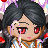••••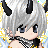•••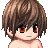••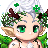Report | 03/03/2016 1:28 pmhello smile
Report | 08/18/2014 7:40 amApologies for being late but I still would like to wish you another happy birthday and best wishes to you for another year.
Report | 08/17/2013 9:47 amHappy birthday wherever you are I miss you very much
Report | 08/17/2012 8:30 pmHello and happy birthday,I wish you the best and hope all your dreams come true.
Report | 12/25/2011 10:38 pmHey you, ya you! I miss ur awesome-ness!
Report | 10/25/2011 4:05 pmyou want 100000000 gold then copy/paste this on ten gaia profiles and press f4 then log out and log back in and check your gold!!!!!!!it really works!!!!!!!!!
Report | 08/30/2011 10:25 pmHellooooooooooooo there!
Report | 08/17/2011 8:58 pmHey birthday girl here to send you lots of birthday love and hope all your wishes come true
Report | 06/21/2011 10:09 pmhi, i seen we had a friend in common so figured i would take a look at your profile. lovely picture you have, if i do say so myself. anyways, how's it goin?
Report | 06/03/2011 9:04 pmhey wanan meet up pm me halley

## Forums

Posts per Day: 0.00

Total Posts: 8

Latest Posts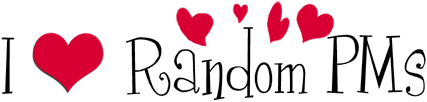my favorite (: <3